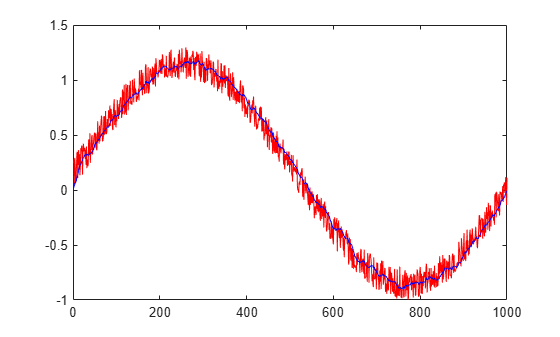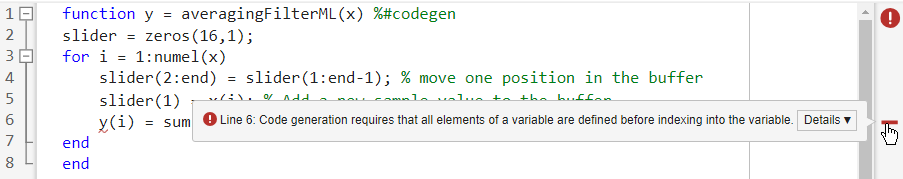# Generate Code for an Averaging Filter

This example shows the recommended workflow for generating C code from a MATLAB® function using the `codegen` command. These are the steps:

1. Add the `%#codegen` directive to the MATLAB function to indicate that it is intended for code generation. This directive also enables the MATLAB code analyzer to identify warnings and errors specific to MATLAB for code generation.

2. Generate a MEX function to check that the MATLAB code is suitable for code generation. If errors occur, you should fix them before generating C code.

3. Test the MEX function in MATLAB to ensure that it is functionally equivalent to the original MATLAB code and that no run-time errors occur.

4. Generate C code.

5. Inspect the C code.

### Prerequisites

There are no prerequisites for this example.

### About the `averaging_filter` Function

The `averaging_filter.m` function acts as an averaging filter on the input signal; it takes an input vector of values and computes an average for each value in the vector. The output vector is the same size and shape as the input vector.

`type averaging_filter`
```% y = averaging_filter(x) % Take an input vector signal 'x' and produce an output vector signal 'y' with % same type and shape as 'x' but filtered. function y = averaging_filter(x) %#codegen % Use a persistent variable 'buffer' that represents a sliding window of % 16 samples at a time. persistent buffer; if isempty(buffer) buffer = zeros(16,1); end y = zeros(size(x), class(x)); for i = 1:numel(x) % Scroll the buffer buffer(2:end) = buffer(1:end-1); % Add a new sample value to the buffer buffer(1) = x(i); % Compute the current average value of the window and % write result y(i) = sum(buffer)/numel(buffer); end ```

The `%#codegen` compilation directive indicates that the MATLAB code is intended for code generation.

### Create Some Sample Data

Generate a noisy sine wave and plot the result.

```v = 0:0.00614:2*pi; x = sin(v) + 0.3*rand(1,numel(v)); plot(x, 'red');```### Generate a MEX Function for Testing

Generate a MEX function using the `codegen` command. The `codegen` command checks that the MATLAB function is suitable for code generation and generates a MEX function that you can test in MATLAB prior to generating C code.

`codegen averaging_filter -args {x}`
```Code generation successful. ```

Because C uses static typing, `codegen` must determine the properties of all variables in the MATLAB files at compile time. Here, the `-args` command-line option supplies an example input so that `codegen` can infer new types based on the input types. Using the sample signal created above as the example input ensures that the MEX function can use the same input.

By default, `codegen` generates a MEX function named `averaging_filter_mex` in the current folder. This allows you to test the MATLAB code and MEX function and compare the results.

### Test the MEX Function in MATLAB

Run the MEX function in MATLAB

```y = averaging_filter_mex(x); % Plot the result when the MEX function is applied to the noisy sine wave. % The 'hold on' command ensures that the plot uses the same figure window as % the previous plot command. hold on; plot(y, 'blue');```### Generate C Code

`codegen -config coder.config('lib') averaging_filter -args {x}`
```Code generation successful. ```

### Inspect the Generated Code

The `codegen` command with the `-config coder.config('lib')` option generates C code packaged as a standalone C library. The generated C code is in the `codegen/lib/averaging_filter/` folder. The files are:

`dir codegen/lib/averaging_filter/`
```. averaging_filter_rtw.mk .. averaging_filter_terminate.c .gitignore averaging_filter_terminate.h _clang-format averaging_filter_terminate.o averaging_filter.a averaging_filter_types.h averaging_filter.c buildInfo.mat averaging_filter.h codeInfo.mat averaging_filter.o codedescriptor.dmr averaging_filter_data.c compileInfo.mat averaging_filter_data.h examples averaging_filter_data.o interface averaging_filter_initialize.c rtw_proj.tmw averaging_filter_initialize.h rtwtypes.h averaging_filter_initialize.o ```

### Inspect the C Code for the `averaging_filter.c` Function

`type codegen/lib/averaging_filter/averaging_filter.c`
```/* * File: averaging_filter.c * * MATLAB Coder version : 5.4 * C/C++ source code generated on : 26-Feb-2022 10:44:19 */ /* Include Files */ #include "averaging_filter.h" #include "averaging_filter_data.h" #include "averaging_filter_initialize.h" #include <string.h> /* Variable Definitions */ static double buffer; /* Function Definitions */ /* * Use a persistent variable 'buffer' that represents a sliding window of * 16 samples at a time. * * Arguments : const double x * double y * Return Type : void */ void averaging_filter(const double x, double y) { double dv; int i; int k; if (!isInitialized_averaging_filter) { averaging_filter_initialize(); } /* y = averaging_filter(x) */ /* Take an input vector signal 'x' and produce an output vector signal 'y' * with */ /* same type and shape as 'x' but filtered. */ for (i = 0; i < 1024; i++) { double b_y; /* Scroll the buffer */ memcpy(&dv, &buffer, 15U * sizeof(double)); /* Add a new sample value to the buffer */ b_y = x[i]; buffer = b_y; /* Compute the current average value of the window and */ /* write result */ for (k = 0; k < 15; k++) { double d; d = dv[k]; buffer[k + 1] = d; b_y += d; } y[i] = b_y / 16.0; } } /* * Use a persistent variable 'buffer' that represents a sliding window of * 16 samples at a time. * * Arguments : void * Return Type : void */ void averaging_filter_init(void) { memset(&buffer, 0, 16U * sizeof(double)); } /* * File trailer for averaging_filter.c * * [EOF] */ ```